### Home > PC > Chapter 2 > Lesson 2.3.3 > Problem2-98

2-98.
1. Consider the area under the curve of f(x) =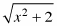on the interval 3 ≤ x ≤ 5. Homework Help ✎

1. Write out the sums you would need to add up to evaluate the right- and left-endpoint rectangle approximations for the area using 4 rectangles. DO NOT add them up. Leave the expression in expanded form.

2. Express each of the sums using summation notation. You should be able to express the different sums by just changing the indices of each summation.

3. Explain how the indices relate to right- and left-endpoint rectangles.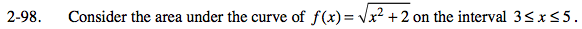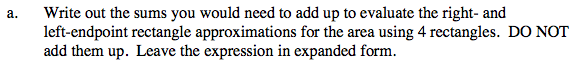Left = (width)(height of each rectangle)

$\text{Left} =(0.5)(\sqrt{3+2}^2+\sqrt{3.5+2}^2+\sqrt{4+2}^2+\sqrt{4.5+2}^2$

$f(x_{0})\ \ \ \ \ \ \ \ \ f(x_{1})\ \ \ \ \ \ \ \ f(x_{2})\ \ \ \ \ \ \ f(x_{3})$

Right = (width)(height of each rectangle)

$\text{Right} =(0.5)(\sqrt{3.5+2}^2+\sqrt{4+2}^2+\sqrt{4.5+2}^2+\sqrt{5+2}^2$

$f(x_{1})\ \ \ \ \ \ \ \ f(x_{2})\ \ \ \ \ \ \ f(x_{3})\ \ \ \ \ \ \ \ \ f(x_{4})$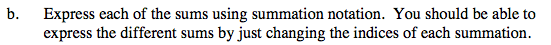$\text{Left endpoints} =\displaystyle\sum\limits_{k=0}^30.5\sqrt{(3+0.5k)^2+2}$

What modification results in the right endpoints?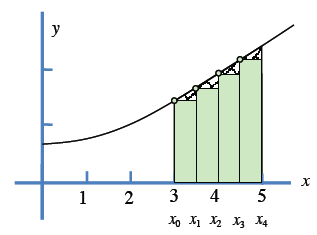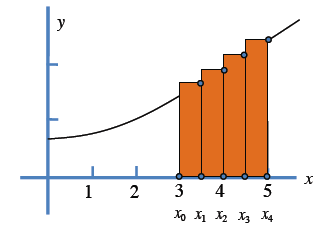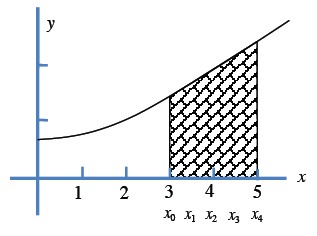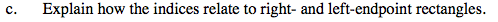The left endpoint indices go from k = 0 to k = 3 whereas the right endpoint indices go from k = 1 to k = 4.